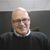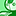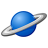# QlikView App Dev

Discussion Board for collaboration related to QlikView App Development.

Announcements
Skip the ticket, Chat with Qlik Support instead for instant assistance.
cancel
Showing results for
Did you mean:Partner - Contributor II

## Set expression problem

Hi All,

Set expression is not working properly ,it is giving zero values.

vperiod-SubField(PeriodWeek,'-',2)

Exp: sum({<Period={"\$(vperiod)"}>}Sales)

Please find the attached app an data.

Muni

15 RepliesPartner - Champion III

To get something that works I believe you have to do this:

Sum( {<UniqueRowID={"=Period=SubField(PeriodWeek,'-',2)"}>} Sales)

The UniqueRowID is as the name implies a field that uniquely identifies each and every row in the table that contains the Sales field.

If you don't have a candidate for that in the table already you will have to create one. It is easy to do in your load script by creating a calculated field in the load statement of the table that contains the Sales field:

SALES:

RowNo() AS Sales.uid,

Sales,

.....

If you do the expression would be changed to:

Sum( {<Sales.uid={"=Period=SubField(PeriodWeek,'-',2)"}>} Sales)Partner - Contributor II
Author

can we get same result with out new fieldCreator III

try this:

vperiod1    =mid(PeriodWeek,6,3)

and

expression as:

if(Match(mid(PeriodWeek,6,3),'03','06','09','12'

),

sum({<Period={"\$(vperiod1)"}>}Sales)/5,

sum({<Period={"\$(vperiod1)"}>}Sales)/4

)Partner - Champion III

As I mentioned - if you already have a field in the table that contains the Sales field that is unique across the rows you can use that and  you don't need a new field.

Look at the Excel data all the rows have the  Period equal to the subfield of the PeriodWeek so I don't understand what you are trying to achieve with this set expression?Partner - Creator III

The set analysis expressions are evaluated once per chart so

{<Period={"\$(vperiod)"}>}

does not work because you want a different value of the variable vperiod for each value of the dimension (PeriodWeek). Why dont you use an IF statement?

If( Match(Only({1<Week>}Right(SubField(PeriodWeek,'-',2),2)),'03','06','09','12'),

sum(if(Period=\$(vperiod), Sales))/5,
sum(if(Period=\$(vperiod), Sales))
/4
)

check the attached App.qwv if you prefer!

Bests,

Jaime.Partner - Contributor II
Author

i have some other filters in the set expression not only period.Partner - Creator III

You can keep that filters except the period and include the IF:

If( Match(Only({1<Week>}Right(SubField(PeriodWeek,'-',2),2)),'03','06','09','12'),

sum( {< OTHER FILTERS>} if(Period=\$(vperiod), Sales))/5,
sum( {< OTHER FILTERS>} if(Period=\$(vperiod), Sales))/4
)Partner - Contributor II
Author

it is not filter the data by above expression.can you please try some other exp.Specialist